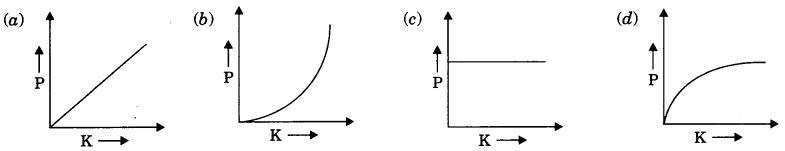# CBSEtips.in

## Saturday, 20 February 2021

### CBSE Class 9 Science - MCQ and Online Tests - Physics - Unit 4 - Work and Energy

#### CBSE Class 9 Science – MCQ and Online Tests – Physics – Unit 4 – Work and Energy

Every year CBSE  schools conducts Annual Assessment exams for 6,7,8,9,11th standards. These exams are very competitive to all the students. So our website provides online tests for all the 6,7,8,9,11th standard’s subjects. These tests are also very effective and useful for those who preparing for any competitive exams like Olympiad etc. It can boost their preparation level and confidence level by attempting these chapter wise online tests.

These online tests are based on latest CBSE syllabus. While attempting these our students can identify the weak lessons and continuously practice those lessons for attaining high marks. It also helps to revise the NCERT textbooks thoroughly.

#### CBSE Class 9 Science – MCQ and Online Tests – Physics – Unit 4 – Work and Energy

Question 1.
Two army persons A and B each of weight of 500 N climb up a rope through a height of 10 m. A takes 20 s while B takes 40 s to achieve this task. What is ratio of the powers of person A and B?
(a) 1 : 2
(b) 1 : 4
(c) 2 : 1
(d) 14 : 1

Answer: (c) 2 : 1

Question 2.
A body is falling from a height h. After it has fallen a height $$\frac{h}{2}$$, it will possess
(a) only potential energy
(b) only kinetic energy
(c) half potential and half kinetic energy
(d) more kinetic and less potential energy

Answer: (c) half potential and half kinetic energy

Question 3.
An iron sphere of mass 10 kg has the same diameter as an aluminium sphere of mass is 3.5 kg. Both spheres are dropped simultaneously from a tower. When they are lo m above the ground, they have the same.
(a) acceleration
(b) momenta
(c) potential energy
(d) kinetic energy

Question 4.
A girl is carrying a school bag of 3 kg mass on her back and moves 200 m on a levelled road. The work done against the gravitational force will be (g = 10 ms²)
(a) 6 × 10³ J
(b) 6 J
(c) 0.6 J
(d) zero

Question 5.
Which one of the following is not the unit of energy?
(a) joule
(b) newton metre
(c) kilowatt
(d) kilowatt hour

Question 6.
Mechanical energy of a body includes
(a) kinetic energy only
(b) potential energy only
(c) kinetic energy and potential energy
(d) none of these

Answer: (c) kinetic energy and potential energy

Question 7.
The work done on an object does not depend upon the
(a) displacement
(b) force applied
(c) angle between force and displacement
(d) initial velocity of the object

Answer: (d) initial velocity of the object

Question 8.
Commercial unit of energy is
(a) joule
(b) kWh
(c) watt
(d) newton

Question 9.
Water stored in a dam possesses
(a) no energy
(b) electrical energy
(c) kinetic energy
(d) potential energy

Answer: (d) potential energy

Question 10.
The number of joules contained in 1 kWh is
(a) 36 × 105 J
(b) 3.6 × 107 J
(c) 36 × 108 J
(d) 3.7 × 107 J

Answer: (a) 36 × 105 J

Question 11.
Potential energy of a body depends on its
(a) position
(b) configuration
(c) position and configuration
(d) mass and velocity

Answer: (c) position and configuration

Question 12.
Which of the following graphs best represents graphical relation between momentum P and kinetic energy K for a body in motion?Question 13.
When a body falls freely towards the earth, then its total energy
(a) increases
(b) decreases
(c) remains constant
(d) first increases and then decreases

Answer: (c) remains constant

Question 14.
A car is accelerated on a levelled road and attains a velocity 4 times of its initial velocity. In this process the potential energy of the car
(a) does not change
(b) becomes twice to that of initial
(c) becomes 4 times that of initial
(d) becomes 16 times that of initial

Answer: (a) does not change

Question 15.
If speed of a car becomes 2 times, its kinetic energy becomes
(a) 4 times
(b) 8 times
(c) 16 times
(d) 12 times

Answer: (a) 4 times

Question 16.
Work done by friction
(a) increases kinetic energy of body
(b) decreases kinetic energy of body
(c) increases potential energy of body
(d) decreases potential energy of body.

Answer: (b) decreases kinetic energy of body

Question 17.
When a coil spring is compressed, the work is done on the spring. The elastic potential energy
(a) increases
(b) decreases
(c) disappears
(d) remains unchanged

Question 18.
In case of negative work the angle between the force and displacement is (NCERT Exemplar)
(a) 0°
(b) 45°
(c) 90°
(d) 180°

Question 19.
One joule work is said to be done when
(a) a force of 1 N displaces a body by 1 cm
(b) a force of 1 N displaces a body by 1 m
(c) a force of 1 dyne displaces a body by 1 m
(d) a force of 1 dyne displaces a body by 1 cm.

Answer: (b) a force of 1 N displaces a body by 1 m

Question 20.
SI unit of power is
(a) watt
(b) joule
(c) newton
(d) metre